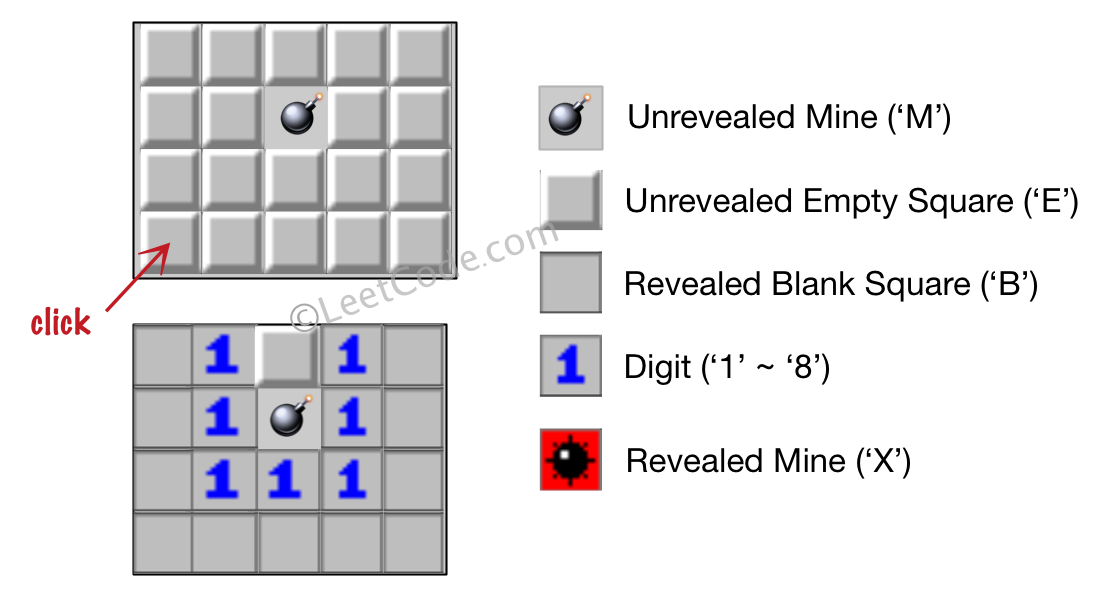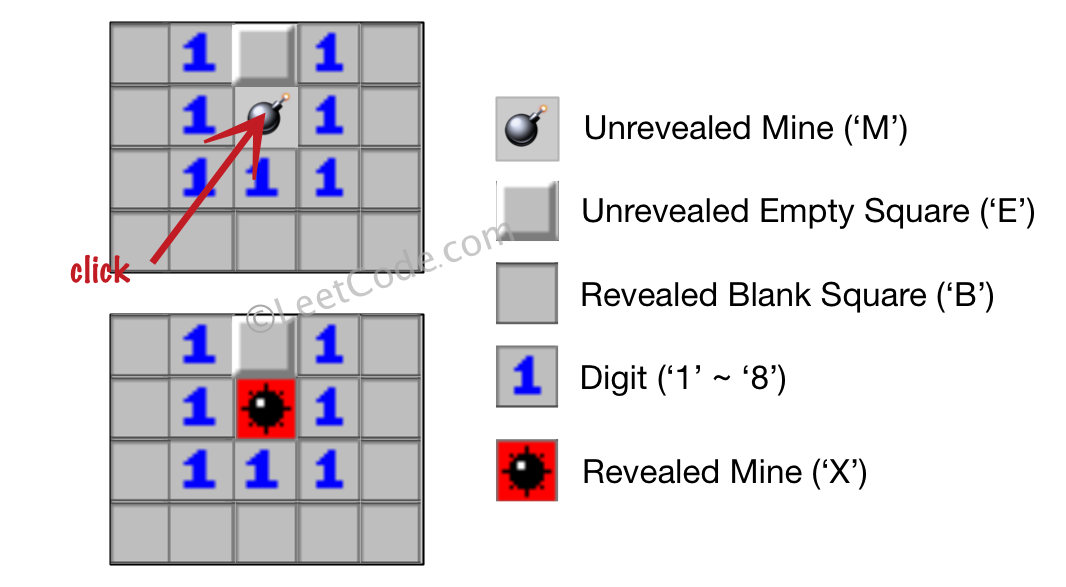##### Welcome to Subscribe On Youtube

Formatted question description: https://leetcode.ca/all/529.html

# 529. Minesweeper

Medium

## Description

Let’s play the minesweeper game (Wikipedia, online game)!

You are given a 2D char matrix representing the game board. ‘M’ represents an unrevealed mine, ‘E’ represents an unrevealed empty square, ‘B’ represents a revealed blank square that has no adjacent (above, below, left, right, and all 4 diagonals) mines, digit (‘1’ to ‘8’) represents how many mines are adjacent to this revealed square, and finally ‘X’ represents a revealed mine.

Now given the next click position (row and column indices) among all the unrevealed squares (‘M’ or ‘E’), return the board after revealing this position according to the following rules:

1. If a mine (‘M’) is revealed, then the game is over - change it to ‘X’.
2. If an empty square (‘E’) with no adjacent mines is revealed, then change it to revealed blank (‘B’) and all of its adjacent unrevealed squares should be revealed recursively.
3. If an empty square (‘E’) with at least one adjacent mine is revealed, then change it to a digit (‘1’ to ‘8’) representing the number of adjacent mines.
4. Return the board when no more squares will be revealed.

Example 1:

Input:

[['E', 'E', 'E', 'E', 'E'],
['E', 'E', 'M', 'E', 'E'],
['E', 'E', 'E', 'E', 'E'],
['E', 'E', 'E', 'E', 'E']]

Click : [3,0]


Output:

[['B', '1', 'E', '1', 'B'],
['B', '1', 'M', '1', 'B'],
['B', '1', '1', '1', 'B'],
['B', 'B', 'B', 'B', 'B']]


Explanation:Example 2:

Input:

[['B', '1', 'E', '1', 'B'],
['B', '1', 'M', '1', 'B'],
['B', '1', '1', '1', 'B'],
['B', 'B', 'B', 'B', 'B']]

Click : [1,2]


Output:

[['B', '1', 'E', '1', 'B'],
['B', '1', 'X', '1', 'B'],
['B', '1', '1', '1', 'B'],
['B', 'B', 'B', 'B', 'B']]


Explanation:Note:

1. The range of the input matrix’s height and width is [1,50].
2. The click position will only be an unrevealed square (‘M’ or ‘E’), which also means the input board contains at least one clickable square.
3. The input board won’t be a stage when game is over (some mines have been revealed).
4. For simplicity, not mentioned rules should be ignored in this problem. For example, you don’t need to reveal all the unrevealed mines when the game is over, consider any cases that you will win the game or flag any squares.

## Solution

First check whether the clicked square is a mine or an empty square. If the clicked square is a mine, change it to ‘X’ and the game is over.

Next deal with the case where the clicked square is an empty square. Do breadth first search starting from the clicked square. If the square has no adjacent mines, for each adjacent cell that is an unrevealed empty square, add it to the search queue and continue searching from the cell in the next iteration. If the square has at least one adjacent mine, then change it to the digit that represents the number of adjacent mines, and stop further searching.

• class Solution {
public char[][] updateBoard(char[][] board, int[] click) {
int row = click, column = click;
if (board[row][column] == 'M')
board[row][column] = 'X';
else if (board[row][column] == 'E')
return board;
}

public void breadthFirstSearch(char[][] board, int row, int column) {
int[][] directions = { {-1, -1}, {-1, 0}, {-1, 1}, {0, 1}, {1, 1}, {1, 0}, {1, -1}, {0, -1} };
int rows = board.length, columns = board.length;
queue.offer(new int[]{row, column});
while (!queue.isEmpty()) {
int[] cell = queue.poll();
int curRow = cell, curColumn = cell;
if (board[curRow][curColumn] != 'E')
continue;
for (int[] direction : directions) {
int newRow = curRow + direction, newColumn = curColumn + direction;
if (newRow >= 0 && newRow < rows && newColumn >= 0 && newColumn < columns) {
char status = board[newRow][newColumn];
if (status == 'M')
}
}
board[curRow][curColumn] = (char) ('0' + adjacentMines);
else {
for (int[] direction : directions) {
int newRow = curRow + direction, newColumn = curColumn + direction;
if (newRow >= 0 && newRow < rows && newColumn >= 0 && newColumn < columns) {
char status = board[newRow][newColumn];
if (status == 'E')
queue.offer(new int[]{newRow, newColumn});
}
}
board[curRow][curColumn] = 'B';
}
}
}
}

• // OJ: https://leetcode.com/problems/minesweeper/
// Time: O(MN)
// Space: O(MN)
class Solution {
public:
vector<vector<char>> updateBoard(vector<vector<char>>& A, vector<int>& click) {
int M = A.size(), N = A.size();
queue<pair<int, int>> q{ { {click, click} } };
while (q.size()) {
auto [x, y] = q.front();
q.pop();
if (A[x][y] == 'M') {
A[x][y] = 'X';
continue;
}
int cnt = 0;
for (int dx = -1; dx <= 1; ++dx) {
for (int dy = -1; dy <= 1; ++dy) {
if (dx == 0 && dy == 0) continue;
int a = x + dx, b = y + dy;
if (a < 0 || a >= M || b < 0 || b >= N) continue;
cnt += A[a][b] == 'M';
}
}
if (cnt) A[x][y] = '0' + cnt;
else {
A[x][y] = 'B';
for (int dx = -1; dx <= 1; ++dx) {
for (int dy = -1; dy <= 1; ++dy) {
if (dx == 0 && dy == 0) continue;
int a = x + dx, b = y + dy;
if (a < 0 || a >= M || b < 0 || b >= N || A[a][b] != 'E') continue;
A[a][b] = '#';
q.emplace(a, b);
}
}
}
}
return A;
}
};

• from collections import deque

class Solution(object):
def updateBoard(self, board, click):
"""
:type board: List[List[str]]
:type click: List[int]
:rtype: List[List[str]]
"""
numbers = "B123456789"
queue = deque([click])
directions = [(-1, 0), (1, 0), (0, -1), (0, 1), (1, 1), (-1, -1), (1, -1), (-1, 1)]
while queue:
i, j = queue.popleft()
if board[i][j] == "B":
continue
if board[i][j] == "M":
board[i][j] = "X"
break
mineCnt = 0
nbrs = []
for di, dj in directions:
ni, nj = i + di, j + dj
if 0 <= ni < len(board) and 0 <= nj < len(board) and board[ni][nj] in ["M", "E"]:
if board[ni][nj] == "M":
mineCnt += 1
else:
nbrs.append((ni, nj))
if mineCnt == 0:
queue.extend(nbrs)
board[i][j] = numbers[mineCnt]
return board